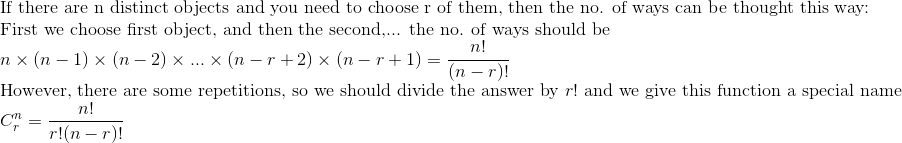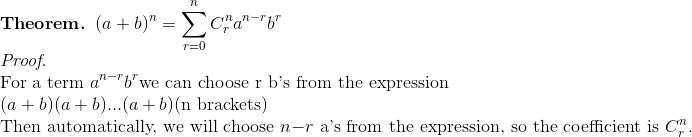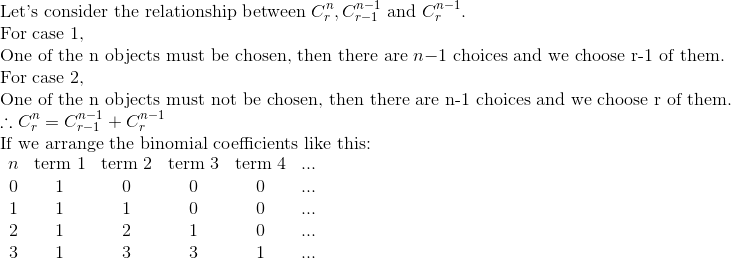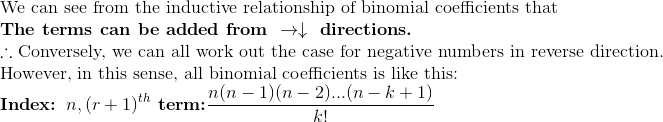Binomial Theorem

There are two 'stages' of binomial theorem: one is the exponent is a positive integer; the other one the exponent is a negative number or fractions.

However, we should all define some basics.Then we can really go into the binomial theorem:By putting different value of a and b, we can have several identities using binomial coefficients. But this is out of scope of this page.

For any positive integer n, we can have the following 'table' of binomial coefficients.Therefore, we can extend the theorem from positive integer to any real number. (According to the Newton's generalisation, we can also make it complex but the things inside the bracket must be real.)